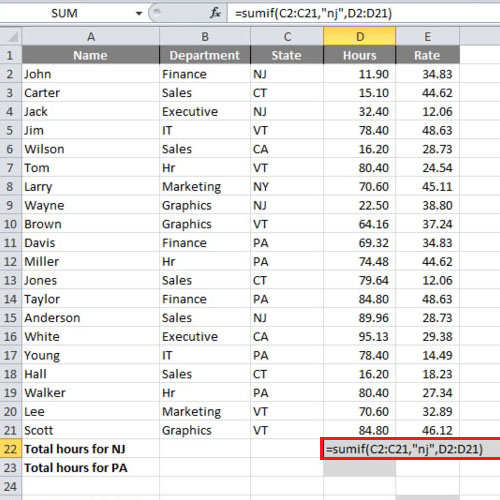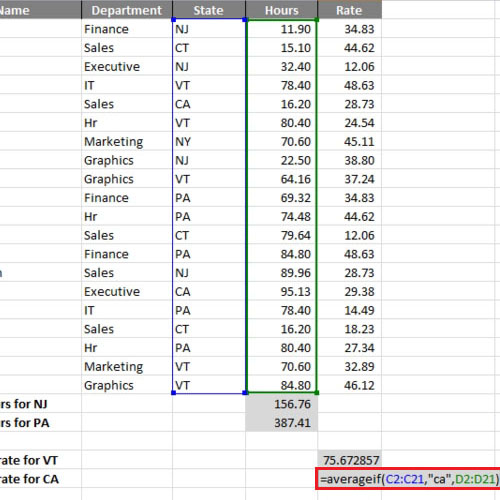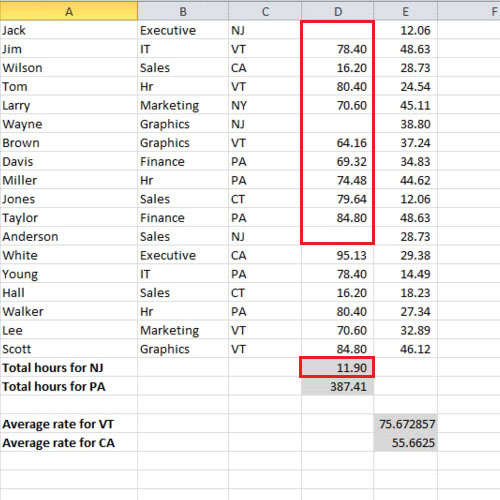## How to Use SUMIF and AVERAGEIF in Excel 2010

MS Excel allows one to use a variety of functions and excel formulas when working with data and carrying out various calculations. You can even choose to test a statement to see if it is true or false. The if function allows you to apply conditional formulas that check the validity of a certain condition and test it for the data range you have applied it on.

Follow this step by step tutorial to learn How to use sumif on excel along with averageif.

Step # 1 – Using the SUMIF function

First of all, we will calculate the total hours for New Jersey. In order to do so, use the sumif on excel and type “=sumif(c2:c21,”nj”,d2:d21)”. This signifies that we want Excel to only take the hours from the state of NJ which is New Jersey and the range is defined already. After typing out the formula, press the “enter” key.Step # 2 – Using the AVERAGEIF function

In this tutorial, we will calculate the average number of hours for the state of Vermont by inserting “=averageif(c2:c21,”vt”,d2:d21)”. Once done, we will press the “enter” key. In order to calculate the average number of hours for the state of “California” we will make a slight adjustment in the function mentioned above. Instead of writing “vt”, we will write “ca”.Step # 3 – Deleting some values

The state of NJ appears four times in the list of the states. If we delete three values from cells “d4”, “d9” and “d15”, only the first value for NJ which is “11.90” will remain.